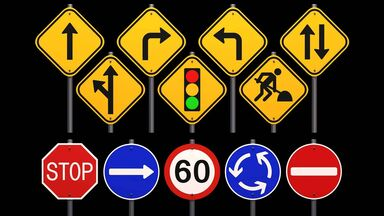# What are Examples of Geometric Shapes in Real Life?

Geometric shapes are everywhere. No matter where you look, almost everything is made up of both two-dimensional (2D) and three-dimensional (3D) geometric shapes. Keep reading for real-life geometric shape examples that make up the world around us.geometric shapes examples in street signs

## Examples of 2D Geometric Shapes

Two-dimensional shapes are flat figures that have width and height, but no depth. Circles, squares, triangles, and rectangles are all types of 2D geometric shapes. Check out a list of different 2D geometric shapes, along with a description and examples of where you can spot them in everyday life.

Keep in mind that these shapes are all flat figures without depth. That means you can take a picture of these items and you can still determine their shape. The same isn't true for three-dimensional shapes.

### Circle Examples

A circle is a round shape with the same radius from a fixed point in the center. Examples of circles in real life include:

• pizza pies
• wheels of a bike
• clock faces
• dinner plates

### Square Examples

Four equal straight sides with four right angles make a square. Some real-life examples of squares are:

• square rubber stamps
• square tiles on the floor
• square paper napkins
• chess boards
• virtual keyboard keys

### Triangle Examples

Triangles are three-sided figures with straight sides. There are lots of different types of triangles, depending on their angles. Examples of triangles in real life are:

• slices of pizza
• a sandwich cut diagonally
• some tortilla chips
• sails on a boat
• yield traffic signs

### Rectangle Examples

Like a square, a rectangle has four straight sides with four right angles. However, two sides are shorter than the other two sides. Check out these examples of real-life rectangles:

• chapter book covers
• cell phones
• some picture frames
• dollar bills
• some ice cream sandwiches

### Pentagon Examples

Pentagons are shapes with five sides of equal length. They are less common than quadrilaterals, which have four sides, but can still be found in real life. Some examples of pentagons are:

• The Pentagon building
• black sections on soccer balls
• home plate in baseball
• traffic crossing signs

### Hexagon Examples

When you add one side to a pentagon, you get a hexagon. A hexagon has six straight sides of equal length. Check out these examples of real-life hexagons:

• ice crystals
• snowflakes
• beehive cells
• outline of a metal nut
• white sections on soccer balls

### Octagon Examples

Eight straight sides, typically of equal length, make an octagon. They are more common in real life than you may think. Some examples of octagons are:

• stop signs
• open umbrellas
• UFC ring
• poker tables

### Trapezoid Examples

A trapezoid is a four-sided figure with just one pair of parallel sides. You can find trapezoids in the following examples:

• the trusses on bridges
• some handbag sides
• a half-eaten piece of pizza
• musical dulcimer

## Examples of 3D Geometric Shapes

Unlike two-dimensional shapes, three-dimensional shapes have width, height and depth. Examples of 3D shapes include pyramids, spheres and cubes. Take a look at these everyday 3D geometric shape examples.

Some of these shapes are interchangeable, of course. For example, a bag might not always be a parallelogram, as there are certainly circular bags and other types possible. This list is also not exhaustive either, as there are many other two-dimensional and three-dimensional geometric shapes.

### Sphere Examples

Spheres are round solid figures. Like circles, they have a radius in the center that is equidistant to every point on the sphere. However, unlike circles, they have volume and depth. Examples of real-life spheres are:

• planets
• oranges
• marbles
• tennis balls

### Cube Examples

Each of the six faces of a cube is a square. When they are put together in a 3D cube shape, also known as a square prism, they create depth. Some examples of cubes include:

• six-sided dice
• sugar cubes
• square building blocks
• Rubix cubes
• square packing boxes

### Cone Examples

A cone has a circular base that tapers to one point. Cones are very common in everyday items, including:

• traffic cones
• waffle ice cream cones
• party hats
• Christmas trees
• funnels

### Cylinder Examples

Cylinders are three-dimensional figures with parallel sides and a circular cross-section. Some real-life examples of cylinders are:

• cardboard paper towel tube
• straight pipes
• drinking glasses
• chapstick tubes
• cans

### Ellipsoid Examples

An ellipsoid is a sphere that is not perfectly round because it has been flattened at two sides. Also known as a spheroid, an ellipsoid creates a 3D oval effect. Some examples of ellipsoids are:

• footballs
• eggs
• some of Saturn's satellite moons (Mimas, Enceladus, Tethys)

### Rectangular Prism Examples

A rectangular prism is a 3D figure where one pair of opposite sides are the same shape, connected by straight, parallel sides. They have four rectangular faces and two square faces. You can find rectangular prisms in these examples:

• sticks of butter
• bricks
• cameras
• cereal boxes
• rectangular packing boxes

### Triangular Prism

Triangular prisms are just like rectangular prisms, except that their square faces are triangles, making them three-sided prisms. Examples of real-life triangular prisms include:

• tents
• wedges of watermelon
• wedges of cheese
• slices of cake

### Pyramid Examples

A three-dimensional figure with one flat side and edges emerging to come together at a point is a pyramid. They can have any shape with three or more sides as their base, including a triangle (triangular pyramid), square (square pyramid) and pentagon (pentagonal pyramid). Examples include:

• the Great Pyramid of Giza
• the roof of a house
• some free-standing cheese graters
• glass Lourve Pyramid